## GATE 2006

 Question 1

Consider the polynomial p(x) = a0 + a1x + a2x2 + a3x3, where ai ≠ 0, ∀i. The minimum number of multiplications needed to evaluate p on an input x is:

 A 3 B 4 C 6 D 9
Engineering-Mathematics       Numerical-Methods
Question 1 Explanation:
Given polynomial equation is
p(x) = a0 + a1x + a2x2 + a3x3 where ai≠0
This can be written as
p(x) = a0 +x( a1 + a2x + a3x2)=a0+(a1+(a2+a3x)x)x
Total no. of multiplications required is 3
i.e., a3x = K.....(i)
(a2+K)x = M..... (ii)
(a1+M)x=N...... (iii)
 Question 2

Let X, Y, Z be sets of sizes x, y and z respectively. Let W = X×Y and E be the set of all subsets of W. The number of functions from Z to E is:

 A Z2xy B Z×2xy C Z2x+y D 2xyz
Engineering-Mathematics       Sets-And-Functions
Question 2 Explanation:
A set consists of n elements then no. of possible subsets are 2n.
A set ‘P’ consists of m elements and ‘Q’ consists of n elements then total number of function from P to Q is mn.
⇒ E be the no. of subsets of W = 2|w| = 2|xxy| = 2xy
No. of function from Z to E is = (2xy)z = (2xy)z = 2xyz
 Question 3

The set {1, 2, 3, 5, 7, 8, 9} under multiplication modulo 10 is not a group. Given below are four plausible reasons. Which one of them is false?

 A It is not closed B 2 does not have an inverse C 3 does not have an inverse D 8 does not have an inverse
Engineering-Mathematics       Sets-And Relation
Question 3 Explanation:
The given set is x = {1,2,3,5,7,8,9}
Option A:
It is not closed under multiplication. After multiplication modulo (10) we get ‘0’. The ‘0’ is not present in the set.
(2*5)%10 ⇒ 10%10 = 0
Option B:
2 does not have an inverse such as
(2*x)%10 ≠ 1
Option C:
3 have an inverse such that
(3*7)%10 = 1
Option D:
8 does not have an inverse such that
(8*x)%10 ≠ 1
 Question 4

A relation R is defined on ordered pairs of integers as follows: (x,y) R(u,v) if x < u and y > v. Then R is: Then R is:

 A Neither a Partial Order nor an Equivalence Relation B A Partial Order but not a Total Order C A Total Order D An Equivalence Relation
Question 4 Explanation:
If a relation is equivalence then it must be
i) Symmetric
ii) Reflexive
iii) Transitive
If a relation is partial order relation then it must be
i) Reflexive
ii) Anti-symmetric
iii) Transitive
If a relation is total order relation then it must be
i) Reflexive
ii) Symmetric
iii) Transitive
iv) Comparability
Given ordered pairs are (x,y)R(u,v) if (xv).
Here <, > are using while using these symbol between (x,y) and (y,v) then they are not satisfy the reflexive relation. If they uses (x<=u) and (y>=u) then reflexive relation can satisfies.
So, given relation cannot be a Equivalence. Total order relation or partial order relation.
 Question 5

For which one of the following reasons does Internet Protocol (IP) use the time-to- live (TTL) field in the IP datagram header?

 A Ensure packets reach destination within that time B Discard packets that reach later than that time C Prevent packets from looping indefinitely D Limit the time for which a packet gets queued in intermediate routers
Computer-Networks       IP-Header
Question 5 Explanation:
It prevent infinite looping over network . Because each router decrease its value by one and when this (TTL) value become zero , then it is discarded by router silently.
 Question 6

Consider three CPU-intensive processes, which require 10, 20 and 30 time units and arrive at times 0, 2 and 6, respectively. How many context switches are needed if the operating system implements a shortest remaining time first scheduling algorithm? Do not count the context switches at time zero and at the end.

 A 1 B 2 C 3 D 4
Operating-Systems       Process-Scheduling
Question 6 Explanation:Total no.of context switches is 2.
 Question 7

Consider the following grammar.

```   S → S * E
S → E
E → F + E
E → F
F → id ```

Consider the following LR(0) items corresponding to the grammar above.

```(i) S → S * .E
(ii) E → F. + E
(iii) E → F + .E ```

Given the items above, which two of them will appear in the same set in the canonical sets-of-items for the grammar?

 A (i) and (ii) B (ii) and (iii) C (i) and (iii) D None of the above
Compiler-Design       Parsers
Question 7 Explanation:
As we can see in the below given LR(0) items, that all three belongs to different state (sets).Question 8

You are given a free running clock with a duty cycle of 50% and a digital waveform f  which changes only at the negative edge of the clock. Which one of the following circuits (using clocked D flip-flops) will delay the phase of f  by 180°?

 ABCDDigital-Logic-Design       Sequential-Circuits
Question 8 Explanation:
Duty cycle is the period of time where the signal high, i.e. 1.
50% of duty cycle means, the wave is 1 for half of the time and 0 for the other half of the time. It is a usual digital signal with 1 and 0.
The waveform f changes for every negative edge, that means f value alters from 1 to 0 or 0 to 1 for every negative edge of the clock.
Now the problem is that we need to find the circuit which produces a phase shift of 180, which means the output is 0 when f is 1 and output is 1 when f is 0.
Like the below image.Now to find the answer we can choose elimination method.
F changes for negative edge, so that output too should change at negative edge. i.e if f becomes 0, then at the same time output should become 1, vice versa.
So, whenever input changes, at the same point of time output too should change. As input changes on negative edge, the output should be changed at negative edge only.
To have the above behaviour, the second D flip-flop which produces the final output should be negative edge triggered. because whatever the 2nd flip-flop produces, that is the output of the complete circuit.
So, we can eliminate option a, d.
Now either b or c can be answer.
How the flip-flop chain works in option b and c is as below.
—> F changes at negative edge.
—> But flip-flop1 responds at next positive edge.
—> After this flip-flop2 responds at next negative edge.
That means flip-flop2 produces the same input which is given to flip-flop now after a positive edge and a negative edge, that means a delay of one clock cycle, which is 180 degrees phase shift for the waveform of f.
Option b) we are giving f’, so that the output is f’ with 180 degrees phase shift.
Option c) we are giving f, so that the output is f with 180 degrees phase shift.
Hence option C is the answer.
 Question 9

A CPU has 24-bit instructions. A program starts at address 300 (in decimal). Which one of the following is a legal program counter (all values in decimal)?

 A 400 B 500 C 600 D 700
Computer-Organization       Machine-Instructions
Question 9 Explanation:
The instruction is of 24 bits or 3 bytes. Now the program starts at address 300 and the next will be 303 then, 306, then 309 and so on.
So finally we can say that the values in the program counter will always be the multiple of 3.
Hence, option (C) is correct.
 Question 10

In a binary max heap containing n numbers, the smallest element can be found in time

 A O(n) B O(log n) C O(log log n) D O(1)
Data-Structures       Heap-Tree
Question 10 Explanation:
In a MAX heap the smallest values of the heap will always be present on the last level of heap and time complexity of reaching the last level of heap is O(n).
We have to search all the elements to reach the smallest element and heap using linear search.
To traverse all elements using linear search will take O(n) time.
 Question 11
Consider a weighted complete graph G on the vertex set {v1, v2, ..., vn} such that the weight of the edge (vi, vj) is 2|i - j|. The weight of a minimum spanning tree of G is:
 A n-1 B 2n-2 CD n2
Algorithms        Minimum-Spanning-Tree
Question 11 Explanation:
1) Including the initial call which means write the length of the spanning tree, a simple one it is. (Based on a particular graph)
2) Weight of the minimum spanning tree = 2|2 - 1| + 2|3 - 2| + 2|4 - 3| + 2|5 - 4| .... + 2| n - (n-1) | = 2n - 2
 Question 12

To implement Dijkstra’s shortest path algorithm on unweighted graphs so that it runs in linear time, the data structure to be used is:

 A Queue B Stack C Heap D B-Tree
Algorithms        ACPath
Question 12 Explanation:
Queue: Basically we do BFS-traversal of the graph to get the shortest paths. There is no point of using Min-heap if it is unweighted graph. Even though if you use after every extract min operation you have to do min-heapify which takes O(log V) time. so, the total time will be O(VlogV). As it is undirected graph you should do BFS from the source then you will get shortest path only. It will take O(V+E) time.
 Question 13

A scheme for storing binary trees in an array X is as follows. Indexing of X starts at 1 instead of 0. The root is stored at X. For a node stored at X[i], the left child, if any, is stored in X[2i] and the right child, if any, in X[2i+1]. To be able to store any binary tree on n vertices the minimum size of X should be.

 A log2n B n C 2n+1 D 2n-1
Data-Structures       Binary-Trees
Question 13 Explanation:
The binary right skewed tree follows 2n -1 because level 2 value is 7 and level 3 value 15.
Note: Assume level numbers are start with 0.
 Question 14

Which one of the following in place sorting algorithms needs the minimum number of swaps?

 A Quick sort B Insertion sort C Selection sort D Heap sort
Algorithms        Sorting
Question 14 Explanation:
Selection sort requires minimum number of swaps i.e O(n). The algorithm finds the minimum value, swaps it with the value in the first position, and repeats these steps for the remainder of the list. It does no more than n swaps, and thus is useful where swapping is very expensive.
 Question 15

Consider the following C-program fragment in which i, j and n are integer variables.

` for (i = n, j = 0; i >0; i /= 2, j += i);  `

Let val(j) denote the value stored in the variable j after termination of the for loop. Which one of the following is true?

 A val(j) = θ(logn) B val(j) = θ(√n) C val(j) = θ(n) D val(j) = θ(n logn)
Algorithms        Time-Complexity
Question 15 Explanation:
The loop following series is n/2 + n/4 + n/8 + ... + 1 = Θ(n).
 Question 16

Let S be an NP-complete problem and Q and R be two other problems not known to be in NP. Q is polynomial time reducible to S and S is polynomial-time reducible to R. Which one of the following statements is true?

 A R is NP-complete B R is NP-hard C Q is NP-complete D Q is NP-hard
Algorithms        P-NP
Question 16 Explanation:
NP-Hard- if it can be solved in polynomial time then all NP-Complete can be solved in polynomial time. If NP Hard problem is reducible to another problem in Polynomial Time, then that problem is also NP Hard which means every NP problem can be reduced to this problem in Polynomial Time.
 Question 17

An element in an array X is called a leader if it is greater than all elements to the right of it in X. The best algorithm to find all leaders in an array

 A Solves it in linear time using a left to right pass of the array B Solves it in linear time using a right to left pass of the array C Solves it using divide and conquer in time θ(nlogn) D Solves it in time θ(n2)
Algorithms        Time-Complexity
Question 17 Explanation:
Solves it in linear time using a right to left pass of the array will takes time complexity is O(n).
 Question 18

We are given a set X = {x1, .... xn} where xi = 2i. A sample S ⊆ X is drawn by selecting each xi independently with probability pi = 1/2. The expected value of the smallest number in sample S is:

 A 1/n B 2 C √n D n
Engineering-Mathematics       Probability
Question 18 Explanation:
The smallest element in sample S would be xi for which i is the smallest.
The given probability Pi is for selection of each item independently with probability 1/2.
Now, Probability for x1 to be smallest in S = 1/2
Now, Probability for x2 to be smallest in S = Probability of x1 not being in S × Probability of x2 being in S
= 1/2 × 1/2
Similarly, Probability xi to be smallest = (1/2)i
Now the Expected value isQuestion 19

Let L1 = {0n+m1n0m|n,m ≥ 0}, L2 = {0n+m1n+m0m|n,m ≥ 0}, and L3 = {0n+m1n+m0n+m|n,m ≥ 0}. Which of these languages are NOT context free?

 A L1 only B L3 only C L1 and L2 D L2 and L3
Theory-of-Computation       Context-Free-Language
Question 19 Explanation:
L1 can be accepted by PDA, we need to push all 0’s before 1’s and when 1’s comes in input string we need to pop every 0’s from stack for every 1’s and then for every 0’s. If stack and input string is empty at the same time then the string belongs to L1.
But for L2 and L3 PDA implementation is not possible. The reason is, in L2 there are two comparison at a time, first the number of 0’s in beginning should be equal to 1’s and then 0’s after 1’s must be less than or equal to number of 1’s. Suppose for initial 0’s and 1’s are matched by using stack then after matching stack will become empty and then we cannot determine the later 0’s are equal to or less than number of 1’s. Hence PDA implementation is not possible. Similarly L3 also has the similar reason.
 Question 20

Consider the following log sequence of two transactions on a bank account, with initial balance 12000, that transfer 2000 to a mortgage payment and then apply a 5% interest.

```
1. T1 start
2. T1 B old=12000 new=10000
3. T1 M old=0 new=2000
4. T1 commit
5. T2 start
6. T2 B old=10000 new=10500
7. T2 commit
```

Suppose the database system crashes just before log record 7 is written. When the system is restarted, which one statement is true of the recovery procedure?

 A We must redo log record 6 to set B to 10500. B We must undo log record 6 to set B to 10000 and then redo log records 2 and 3. C We need not redo log records 2 and 3 because transaction T1 has committed. D We can apply redo and undo operations in arbitrary order because they are idempotent.
Database-Management-System       Transactions
Question 20 Explanation:
When the database system crashes after the transactions have committed then we need to redo the log records. And if the database system crashes before the transactions have committed then we need to undo the log records.
So from above theory we can say that option (B) is the correct answer.
 Question 21

For each element in a set of size 2n, an unbiased coin is tossed. The 2n coin tosses are independent. An element is chosen if the corresponding coin toss were head. The probability that exactly n elements are chosen is:

 ABCD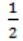Engineering-Mathematics       Probability
Question 21 Explanation:
Total number of coins = 2n
No. of elements selected = n
Probability of getting head = ½
Probability of n heads out of 2n coin tosses is
2nCn*(1/2)n*(1/2)n = 2nCn/4n
 Question 22

Let E, F and G be finite sets.

Let X = (E∩F) - (F∩G) and Y = (E - (E∩G)) - (E-F).

Which one of the following is true?

 A X ⊂ Y B X ⊃ Y C X = Y D X - Y ≠ ∅ and Y - X ≠ ∅
Engineering-Mathematics       Sets-And-Relation
Question 22 Explanation:
Let us consider
E = {(1,1), (1,2), (1,3), (2,2), (2,3), (3,3), (3,1)}
F = {(1,1), (2,2), (3,3)}
G = {(1,3), (2,1), (2,3), (3,1)}
X = (E∩F) - (F∩G)
= {(1,1), (2,2), (3,3) - ∅}
= {(1,1), (2,2), (3,3)} (✔️)
Y = (E - (E∩G) - (E - F))
= (E - {(1,3), (2,3), (3,1)} - {(1,2), (1,3), (2,3), (3,1)})
= {(1,1), (1,2), (1,3), (2,2), (2,3), (3,3), (3,1)} - {(1,3), (2,2), (2,1)} - (1,2), (1,3), (2,3), (3,1)}
= {(1,1), (1,2), (2,2), (3,3)} - {(1,2), (1,3), (2,3), (3,1)}
= {(1,1), (2,2), (3,3)} (✔️)
X = Y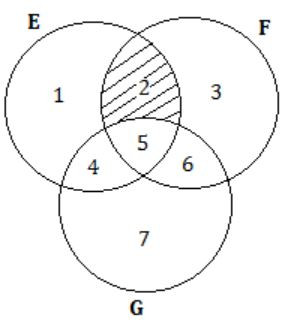X = (E∩F) - (F∩G) = {2,5} - {5} = {2}
Y = (E - (E∩G) - (E - F))
= {(1,2,4,5) - (4,5) - (1,4)}
= {(1,2) - (1,4)}
= {2}
X = Y
 Question 23

F is an n×n real matrix. b is an n×1 real vector. Suppose there are two n×1 vectors, u and v such that, u≠v and Fu=b, Fv=b. Which one of the following statements is false?

 A Determinant of F is zero B There are an infinite number of solutions to Fx=b C There is an x≠0 such that Fx=0 D F must have two identical rows
Engineering-Mathematics       Linear-Algebra
Question 23 Explanation:
⇾ Fu = b, Fv = b
Fu = Fv
Fu - Fv = 0
F(u - v) = 0
Given u ≠ v
F = 0 (i.e., Singular matrix, so determinant is zero)
Option A is true.
⇾ Fx = b; where F is singular
It can have no solution (or) infinitely many solutions.
Option B is true.
⇾ x ≠ 0 such that Fx = 0 is True because F is singular matrix (“stated by singular matrix rules). Option C is true.
⇾ F can two identical columns and rows.
Option D is false.
 Question 24

Given a set of elements N = {1, 2, ..., n} and two arbitrary subsets A⊆N and B⊆N, how many of the n! permutations π from N to N satisfy min(π(A)) = min(π(B)), where min(S) is the smallest integer in the set of integers S, and π(S) is the set of integers obtained by applying permutation π to each element of S?

 A (n-|A ∪ B|) |A| |B| B (|A|2+|B|2)n2 C n!(|A∩B|/|A∪B|) DEngineering-Mathematics       Combinatorics
Question 24 Explanation:
Given a set of elements N = {1, 2, 3, ...N}
Two arbitrary subsets A⊆N and B⊆N.
Out of n! permutations π from N to N, to satisfy
min(π(A)) = min (π(B))
*) π(S) is the set of integers obtained by applying permutation π to each element of S.
If min(π(A)) =min (π(B)), say y = π(x) is the common minimum.
Since the permutation π is a 1-to-1 mapping of N,
x ∈ A∩B
∴ A∩B cannot be empty.
⇒ y = π(x)
= π(A∩B) is the minimum of π(A∪B) is the minimum of π(A) and π(B) are to be same.
You can think like
*) If the minimum of π(A) and π(B) are same [min π(A)] = min [π(B)]
then min(π(A∩B)) = min(π(A∪B))
∴ Total number is given by n! |A∩B|/|A∪B|
*) Finally
Considering all possible permutations, the fraction of them that meet this condition |π(A∩B)| / |π(A∪B)|
[The probability of single permutation].
Ex: N = {1, 2, 3, 4} A = {1, 3} B = {1, 2, 4}Since π is one to one mapping
|π(A∩B)| = |A∩B|
∴ π(A) = {1, 2}
π(B) = {1, 4, 3}
π(A∩B) = {1}
π(A∪B) = {1, 2, 3, 4}
4! × 1/4 = 6
 Question 25

Let S = {1,2,3,....,m}, m > 3. Let x1, x2,....xn be the subsets of S each of size 3. Define a function f from S to the set of natural numbers as, f(i) is the number of sets of Xj that contain the element i. That is f(i) = |{j|i ∈ Xj|}|.

Thenis

 A 3m B 3n C 2m+1 D 2n+1
Engineering-Mathematics       Sets And Functions
Question 25 Explanation: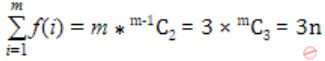Question 26

Which one of the first order predicate calculus statements given below correctly express the following English statement?

`     Tigers and lions attack if they are hungry or threatened.`
 A ∀x [(tiger(x) ∧ lion(x)) → {(hungry(x) ∨ threatened(x)) → attacks(x)}] B ∀x [(tiger(x) ∨ lion(x)) → {(hungry(x) ∨ threatened(x)) ∧ attacks(x)}] C ∀x [(tiger(x) ∨ lion(x)) → {(attacks(x) → (hungry (x)) ∨ threatened (x))}] D ∀x [(tiger(x) ∨ lion(x)) → {(hungry(x) ∨ threatened(x)) → attacks(x)}]
Engineering-Mathematics       Propositional-Logic
Question 26 Explanation:
Tigers and lions attack if they are hungry (or) threatened.
Here we have two cases.
i) If Tiger is hungry (or) threaten that will attack.
ii) If Lion is hungry (or) threaten that will attack.
If Tiger is hungry (or) threaten then both lion and tiger will not attack only Tiger will attack and vice-versa.
Then answer is
∀x[(tiger(x) ∨ lion(x)) → {(hungry(x) ∨ threatened(x)) → attacks(x)}]
Note: Don’t confuse with the statement Tiger and Lion.
 Question 27

Consider the following propositional statements:

P1 : ((A ∧ B) → C)) ≡ ((A → C) ∧ (B → C))
P2 : ((A ∨ B) → C)) ≡ ((A → C) ∨ (B → C))

Which one of the following is true?

 A P1 is a tautology, but not P2 B P2 is a tautology, but not P1 C P1 and P2 are both tautologies D Both P1 and P2 are not tautologies
Engineering-Mathematics       Propositional-Logic
Question 27 Explanation:
It’s better to draw truth table such thatBoth P1 and P2 are not Tautologies.
 Question 28

A logical binary relation ⊙,is defined as follows:Let ~ be the unary negation (NOT) operator, with higher precedence than ⊙. Which one of the following is equivalent to A∧B ?

 A (~A⊙B) B ~(A⊙~B) C ~(~A⊙~B) D ~(~A⊙B)
Engineering-Mathematics       Sets-And-Relation
Question 28 Explanation: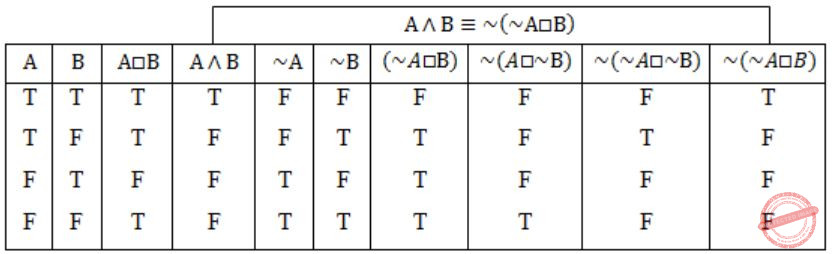Question 29

If s is a string over (0 + 1)* then let n0(s) denote the number of 0’s in s and n1(s) the number of 1’s in s. Which one of the following languages is not regular?

 A L = {s ∈ (0+1)* | n0(s) is a 3-digit prime} B L = {s ∈ (0+1)* | for every prefix s' of s,|n0(s') - n1(s')| ≤ 2} C L = {s ∈ (0+1)* |n0(s) - n1(s)| ≤ 4} D L = {s ∈ (0+1)* | n0(s) mod 7 = n1(s) mod 5 = 0}
Theory-of-Computation       Regular-Language
Question 29 Explanation:
Since 3-digit prime numbers are finite so language in option A is finite, hence it is regular.
Option B: The DFA contains 6 states
State 1: n0(s') - n1(s') = 0
State 2: n0(s') - n1(s') = 1
State 3: n0(s') - n1(s') = 2
State 4: n0(s') - n1(s') = -1
State 5: n0(s') - n1(s') = -2
State 6: Dead state (trap state)
Hence it is regular.
Option D: Product automata concept is used to construct the DFA.
mod 7 has remainders {0,1,2,3,4,5,6} and mod 5 remainders {0,1,2,3,4}
So product automata will have 35 states.
But option C has infinite comparisons between number of 0’s and 1’s.
For ex: n0(s) = 5 and n1(s) = 1 then n0(s) - n1(s) = 4 and if n0(s) = 15 and n1(s) = 11 then n0(s) - n1(s) = 4.
Hence this is CFL.
 Question 30

For S ∈ (0+1) * let d(s) denote the decimal value of s (e.g. d(101) = 5). Let L = {s ∈ (0+1)*|d(s)mod5 = 2 and d(s)mod7 = 4}.

Which one of the following statements is true?

 A L is recursively enumerable, but not recursive B L is recursive, but not context-free C L is context-free, but not regular D L is regular
Theory-of-Computation       Identify-Class-Language
Question 30 Explanation:
Let L1 = {s ∈ (0+1)* | d(s) mod5 = 2}, we can construct the DFA for this which will have 5 states (remainders 0,1,2,3,4)
L2 = {s ∈ (0 + 1)* | d(s) mod7 = 4}, we can construct the DFA for this which will have 7 states (remainders 0,1,2,3,4,5,6)
Since L1 and L2 have DFAs, hence they are regular. So the resulting Language.
L = L1 ∩ L2 (compliment) must be regular (by closure properties, INTERSECTION of two regular languages is a regular language).
 Question 31

Let SHAM3 be the problem of finding a Hamiltonian cycle in a graph G = (V,E) with |V| divisible by 3 and DHAM3 be the problem of determining if a Hamiltonian cycle exists in such graphs. Which one of the following is true?

 A Both DHAM3 and SHAM3 are NP-hard B SHAM3 is NP-hard, but DHAM3 is not C DHAM3 is NP-hard, but SHAM3 is not D Neither DHAM3 nor SHAM3 is NP-hard
Algorithms        P-NP
Question 31 Explanation:
Whether to finding a Hamiltonian cycles exist (or) not is a NP Hard problem. So both DHAM3 and SHAM3 are NP-hard.
 Question 32

Consider the following statements about the context free grammar

`G = {S → SS, S → ab, S → ba, S → ε} `
I. G is ambiguous
II. G produces all strings with equal number of a’s and b’s
III. G can be accepted by a deterministic PDA.

Which combination below expresses all the true statements about G?

 A I only B I and III only C II and III only D I, II and III
Theory-of-Computation       Contest-Free-Grammar
Question 32 Explanation:
Is ambiguous, as it has two parse tree for the string “abbaba”G doesn’t product all strings of equal number of a’s and b’s, for ex: string “aabb” doesn’t generate by grammar G.
The language generated by G can be accepted by DPDA. We can notice that grammar G generates, a’s and b’s in pair, i.e. either “ab” or “ba”, so the strings in language are {ab, ba, abab, abba, baba, ….}
We can design the DPDA:Question 33

Let L1 be a regular language, L2 be a deterministic context-free language and L3 a recursively enumerable, but not recursive, language. Which one of the following statements is false?

 A L1 ∩ L2 is a deterministic CFL B L3 ∩ L1 is recursive C L1 ∪ L2 is context free D L1 ∩ L2 ∩ L3 is recursively enumerable
Theory-of-Computation       Identify-Class-Language
Question 33 Explanation:
Option A is true, as by closure property (R is a regular language and L is any language)
L ∩ R = L ( i.e. L Intersection R is same type as L )
So L1 ∩ L2 is a deterministic CFL.
Option B is false, as L3 is recursive enumerable but not recursive, so intersection with L1 must be recursive enumerable, but may or may not be recursive.
Option C is true, as by closure property (R is a regular language and L is any language)
L U R = L ( i.e. L UNION R is same type as L )
So, L1 ∪ L2 is deterministic context free, hence it is also context free.
Option D is true, as L1 ∩ L2 is DCFL and DCFL ∩ L3 is equivalent to DCFL ∩ Recursive enumerable.
As every DCFL is recursive enumerable, so it is equivalent to Recursive enumerable ∩ Recursive enumerable. And recursive enumerable are closed under INTERSECTION so it will be recursive enumerable.
 Question 34

Consider the regular language L = (111 + 11111)*. The minimum number of states in any DFA accepting this languages is:

 A 3 B 5 C 8 D 9
Theory-of-Computation       Finite-Automata
Question 34 Explanation:
L = (111 + 11111)* generates the strings {ϵ, 111, 11111, 111111, 11111111, …….}
i.e. it generates any string which can be obtained by repetition of three and five 1’s (means length 3, 6, 8, 9, 10, 11, …}
The DFA for the L = (111 + 11111)* is given below.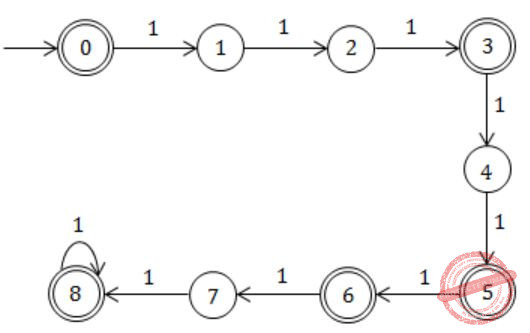Question 35Consider the circuit above. Which one of the following options correctly represents f(x,y,z)?

 AB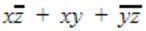CDDigital-Logic-Design       Multiplexer
Question 35 Explanation:
f = yx + y’ (zy’+z’x)
= xy + zy’ + y’z’x
= x(y+y’z’) + zy’
= x(y+z’) + y’z
= xy + xz’ + y’z
 Question 36

Given two three bit numbers a2a1a0 and b2b1b0 and c, the carry in, the function that represents the carry generate function when these two numbers are added is:

 AB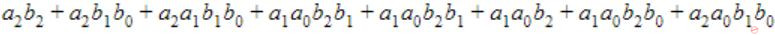CD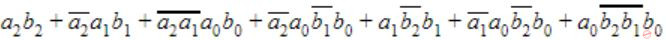Digital-Logic-Design       Carry-Generator
Question 36 Explanation:
Initial Carry c is not included in any option. Hence c=0.
Carry c1 = a0b0
Carry c2 = a2b2 + c1(a2 ⊕ b2 )
= a1b1 +c1 (a1 b’1+ a’1 b1 )
= a1b1 +c1 a1 b’1+ c1 a’1 b1
= (a1b1 + c1a1 b’1)+ (c1 a’1 b1 + a1b1 )
= a1(b1+c1) +b1 (c1 + a1)
= a1b1+b1c1+a1c1
Carry c3 = a2b2 + c2(a2 ⊕ b2)
= a2b2 + c2(a’2b2 + a2b’2 )
= a2b2 + b2c2 + a2c2
= a2b2+a2a1b1+a2a1a0b0+a2a0b1b0+a1b2b1+a1a0b2b0+a0b2b1b0
 Question 37

Consider the circuit in the diagram. The ⊕ operator represents Ex-OR. The D flipflops are initialized to zeroes (cleared).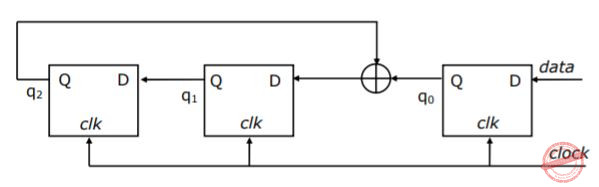The following data: 100110000 is supplied to the “data” terminal in nine clock cycles. After that the values of q2q1q0 are:

 A 000 B 001 C 010 D 101
Digital-Logic-Design       Sequential-Circuits
Question 37 Explanation:
q0N = Data, q1N = q0q22N = q1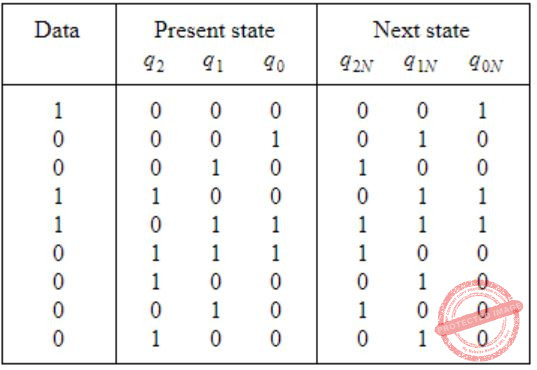Question 38

Consider a Boolean function f(w,x,y,z). Suppose that exactly one of its inputs is allowed to change at a time. If the function happens to be true for two input vectors i1 = 〈w1, x1, y1, z1〉 and i2 = 〈w2, x2, y2, z2〉, we would like the function to remain true as the input changes from vectors i1 to i2 (i1 and i2 differ in exactly one bit position), without becoming false momentarily. Let f(w,x,y,z) = ∑(5,7,11,12,13,15). Which of the following cube covers of f will ensure that the required property is satisfied?

 AB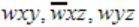CDDigital-Logic-Design       K-Map
Question 38 Explanation:
Static hazard is the situation where, when one input variable changes, the output changes momentarily before stabilizing to the correct value. The most commonly used method to eliminate static hazards is to add redundant logic (consensus terms in the logic expression).
f = X1 * X2 + X1' * X3
If (X1, X2, X3) = (1,1,1) then f=1 because X1 * X2 =1 X1' * X3 = 0.
Let the input is changed from 111 to 011 , then f = 1 because X1 * X2 = 0 X1' * X3 =1.
The output f will be momentarily 0 if AND gate X1 * X2 is faster than the AND gate X1' * X3.
This Hazard can be avoided by adding the term X2 * X3 (because X1 is in true form in first term and in complement form in the second term . So pick the fixed terms X2 and X3 from both terms) to f i.e f = X1 * X2 + X1' * X3 + X2 * X3
Option D is equivalent to f(w, x, y, z) = ∑(5,7,11,12,13,15)
 Question 39

We consider the addition of two 2’s complement numbers bn-1bn-2...b0 and an-1an-2…a0. A binary adder for adding unsigned binary numbers is used to add the two numbers. The sum is denoted by cn-1cn-2c0 and the carry-out by cout. Which one of the following options correctly identifies the overflow condition?

 AB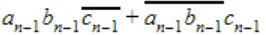C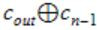D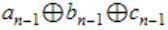Digital-Logic-Design       Carry-Generator
Question 39 Explanation:
There is an overflow if
1. The sign bits are same i.e MSB bits are same.
2. Carry_in ≠ Carry_out.
In option B, the MSB are equal.Question 40

Consider numbers represented in 4-bit gray code. Let h3h2h1h0 be the gray code representation of a number n and let g3g2g1g0 be the gray code of (n+1)(modulo 16) value of the number. Which one of the following functions is correct?

 A g0(h3h2h1h0) = Σ(1,2,3,6,10,13,14,15) B g1(h3h2h1h0) = Σ(4,9,10,11,12,13,14,15) C g2(h3h2h1h0) = Σ(2,4,5,6,7,12,13,15) D g3(h3h2h1h0) = Σ(0,1,6,7,10,11,12,13)
Digital-Logic-Design       Number-Systems
Question 40 Explanation:g2(h3h2h1h0) = Σ(2,4,5,6,7,12,13,15)
 Question 41

A CPU has a cache with block size 64 bytes. The main memory has k banks, each bank being c bytes wide. Consecutive c − byte chunks are mapped on consecutive banks with wrap-around. All the k banks can be accessed in parallel, but two accesses to the same bank must be serialized. A cache block access may involve multiple iterations of parallel bank accesses depending on the amount of data obtained by accessing all the k banks in parallel. Each iteration requires decoding the bank numbers to be accessed in parallel and this takes. k/2 ns The latency of one bank access is 80 ns. If c = 2 and k = 24, the latency of retrieving a cache block starting at address zero from main memory is:

 A 92 ns B 104 ns C 172 ns D 184 ns
Computer-Organization       Cache
Question 41 Explanation:
Cache with block size = 64 bytes
Latency = 80 ns
k = 24
No. of banks are accessed in parallel , then it takes k/2 ns = 24/2 = 12ns
Decoding time = 12ns
Size of each bank C = 2 bytes
Each Bank memory is 2 bytes, and there is 24 banks are there, in one iteration they may get 2*24 = 48 bytes
And getting 64 bytes requires 2 iteration.
T = decoding time + latency = 12+80 = 92
For 2 iterations = 92×2 = 184ns
 Question 42

A CPU has a five-stage pipeline and runs at 1 GHz frequency. Instruction fetch happens in the first stage of the pipeline. A conditional branch instruction computes the target address and evaluates the condition in the third stage of the pipeline. The processor stops fetching new instructions following a conditional branch until the branch outcome is known. A program executes 109 instructions out of which 20% are conditional branches. If each instruction takes one cycle to complete on average, the total execution time of the program is:

 A 1.0 second B 1.2 seconds C 1.4 seconds D 1.6 seconds
Computer-Organization       Pipelining
Question 42 Explanation:
No. of total instructions = 109
20% are condition branches out of 109
⇒ 20/100 × 109
⇒ 2 × 108
In third stage of pipeline it consists of 2 stage cycles.
Total cycle penalty = 2 × 2 × 108 = 4 × 108
Clock speed = 1 GHz
Each Instruction takes 1 cycle i.e., 109 instructions.
Total execution time of a program is
= (109 / 109) +((4× 108) / 109) = 1+0.4 = 1.4 seconds
 Question 43

Consider a new instruction named branch-on-bit-set (mnemonic bbs). The instruction “bbs reg, pos, label” jumps to label if bit in position pos of register operand reg is one. A register is 32 bits wide and the bits are numbered 0 to 31, bit in position 0 being the least significant. Consider the following emulation of this instruction on a processor that does not have bbs implemented.

```temp ← reg & mask
Branch to label if temp is non-zero. ```

The variable temp is a temporary register. For correct emulation, the variable mask must be generated by:

 A mask ← 0×1 << pos B mask ← 0×ffffffff >> pos C mask ← pos D mask ← 0×f
Computer-Organization       Machine-Instructions
Question 43 Explanation:
Using the following operation "temp→reg & mask" we are checking whether bit at position pos in register reg is 1 or not. For that mask should have 1 only in position pos. In all the other positions mask have 0s.
So for mask to have 1 only in position pos and 0s in all the other positions, we can get it by doing left shift on 1, pos number of times.
Out of the given options, in option A this left shift operation on 1 is performed pos number of times. Hence option A is the answer.
 Question 44

Station A uses 32 byte packets to transmit messages to Station B using a sliding window protocol. The round trip delay between A and B is 80 milliseconds and the bottleneck bandwidth on the path between A and B is 128 kbps. What is the optimal window size that A should use?

 A 20 B 40 C 160 D 320
Computer-Networks       Sliding-Window-Protocol
Question 44 Explanation:
Tt = L / B = 32 bytes/ 128 kbps = 32*8/128 ms = 2 ms
Round trip delay = 2 * Tp = 80 ms (given)
Optimal window size is = (Tt + 2*Tp) / Tt = 82 / 2 = 41
Option is not given, closest option is 40.
 Question 45

Two computers C1 and C2 are configured as follows. C1 has IP address 203.197.2.53 and netmask 255.255.128.0. C2 has IP address 203.197.75.201 and netmask 255.255.192.0. Which one of the following statements is true?

 A C1 and C2 both assume they are on the same network B C2 assumes C1 is on same network, but C1 assumes C2 is on a different network C C1 assumes C2 is on same network, but C2 assumes C1 is on a different network D C1 and C2 both assume they are on different networks
Computer-Networks       IP-Address
Question 45 Explanation:
From C1 side,
Subnet mask for C1 is 255.255.128.0.
So it finds the Network ID as,
C1 → 203.197.2.53 AND 255.255.128.0 = 203.197.0.0
C2 → 203.197.75.201 AND 255.255.128.0 = 203.197.0.0
Both same.
From C2 side,
Subnet mask for C2 is 255.255.192.0.
So it finds the network ID as,
C1 → 203.197.2.53 AND 255.255.192.0 = 203.197.0.0
C2 → 203.197.75.201 AND 255.255.192.0 = 203.197.64.0
Both different.
Hence, option 'C' is correct.
 Question 46

Station A needs to send a message consisting of 9 packets to Station B using a sliding window (window size 3) and go-back-n error control strategy. All packets are ready and immediately available for transmission. If every 5th packet that A transmits gets lost (but no acks from B ever get lost), then what is the number of packets that A will transmit for sending the message to B?

 A 12 B 14 C 16 D 18
Computer-Networks       Sliding-Window-Protocol
Question 46 Explanation:
Window size is 3, so maximum 3 packets can be remained unacknowledged. In go back ‘n’ if acknowledge for a packet is not received then packets after that packet is also retransmitted.
Frame sequence for 9 frame is shown below. Frame with bold sequence number gets lost.
1 2 3 4 [5 6 7] 5 6 [7 8 9] 7 8 9 9 = 16
 Question 47

Consider the following graph:Which one of the following cannot be the sequence of edges added, in that order, to a minimum spanning tree using Kruskal’s algorithm?

 A (a—b),(d—f),(b—f),(d—c),(d—e) B (a—b),(d—f),(d—c),(b—f),(d—e) C (d—f),(a—b),(d—c),(b—f),(d—e) D (d—f),(a—b),(b—f),(d—e),(d—c)
Algorithms        Minimum-Spanning-Tree
Question 47 Explanation:
To find Minimum Spanning Tree(MST) using Kruskal’s algorithm we have to follow standard procedure is
1. It follows like forest structure (Means we are disconnecting all the edges connected to vertices).
2. Sort edge weights in Ascending order.
3. Always select the minimum edge weight first according to Spanning Tree rules.
Note: The edge d-e cannot be considered before d-c.
 Question 48

Let T be a depth first search tree in an undirected graph G. Vertices u and n are leaves of this tree T. The degrees of both u and v in G are at least 2. Which one of the following statements is true?

 A There must exist a vertex w adjacent to both u and v in G B There must exist a vertex w whose removal disconnects u and v in G C There must exist a cycle in G containing u and v D There must exist a cycle in G containing u and all its neighbours in G
Data-Structures       Graphs
Question 48 Explanation:
Very difficult assume a graphs here. So, As per the GATE key they given Option D is correct answer.
 Question 49

An implementation of a queue Q, using two stacks S1 and S2, is given below:

```void insert(Q, x) {
push (S1, x);
}

void delete(Q){
if(stack-empty(S2)) then
if(stack-empty(S1)) then {
print(“Q is empty”);
return;
}
else while (!(stack-empty(S1))){
x=pop(S1);
push(S2,x);
}
x=pop(S2);
}```

Let n insert and m(<=n) delete operations be performed in an arbitrary order on an empty queue Q. Let x and y be the number of push and pop operations performed respectively in the process. Which one of the following is true for all m and n?

 A n+m ≤ x < 2n and 2m ≤ y ≤ n+m B n+m ≤ x< 2n and 2m ≤y ≤ 2n C 2m ≤ x< 2n and 2m ≤ y ≤ n+m D 2m ≤ x < 2n and 2m ≤ y ≤ 2n
Data-Structures       Stack-and-Queue
Question 49 Explanation:
Let's first consider for push, i.e., x.
Best case:
First push m elements in S1 then pop m elements from S1 and push them in S2 and then pop all m elements from S2. Now push remaining (n-m) elements to S1.
So total push operation
= m + m + (n-m)
= n+m
Worst Case:
First push all n elements in S1. Then pop n elements from S1 and push them into S2. Now pop m elements from S2.
So total push operation
= n+n
= 2n
Now lets consider for pop operation, i.e., y.
For best case:
First push m elements in S1, then pop m elements and push them in S2. Now pop that m elements from S2. Now push remaining (n-m) elements in S1.
So total pop operation
= m+m
= 2m
For worst case:
First push n elements in S1, then pop them from S1 and push them into S2. Now pop m elements fro m S2.
So total pop operation
= n+m
Therefore, option A is correct answer.
 Question 50

A set X can be represented by an array x[n] as follows:Consider the following algorithm in which x,y and z are Boolean arrays of size n:

```algorithm zzz(x[] , y[], z [])
{
int i;
for (i=O; i<n; ++i)
z[i] = (x[i] ^ ~y[i]) V (~x[i] ^ y[i])
}```

The set Z computed by the algorithm is:

 A (X ∪ Y) B (X ∩ Y) C (X-Y) ∩ (Y-X) D (X-Y) ∪ (Y-X)
Algorithms        Identify-Function
Question 50 Explanation:
X[i] ∧ ~Y[i] can be written as X - Y.
~X[i] ∧ Y[i] can be written as Y - X.
'∨' can be written as Union.
∴ (X-Y) Union (Y-X)
 Question 51

Consider the following recurrence: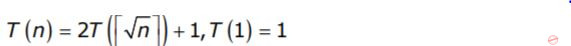Which one of the following is true?

 A T(n) = θ(log logn) B T(n) = θ(logn) C T(n) = θ(√n) D T(n) = θ(n)
Algorithms        Time-Complexity
Question 51 Explanation:
T(n) = 2T(√n)+1
Let n =2m
T(2m) = 2T(2m/2) + 1 --------(1)
Let T(2m) = S(m)
Then equation (1) becomes,
S(m) = 2S(m/2) + 1
So now lets apply Master's theorem,
a=2, b=2, k=0
Since, a>bk, so Case 1 applied hereBut m = log n
So finally the answer is,
O(log n)
 Question 52

The median of n elements can be found in O(n) time. Which one of the following is correct about the complexity of quick sort, in which median is selected as pivot?

 A θ(n) B θ(n logn) C θ(n2) D θ(n3)
Algorithms        Time-Complexity
Question 52 Explanation:
If we choose pivot element element as median then the recurrence relation will be
T(n) = 2T(n/2) +O(n)
The above recurrence in the form of masters theorem: a=2, b=2, k=1, p=0. Since, a=bk, so Case-2 is applied
and also p > -1, soQuestion 53

Consider the following C-function in which a[n] and b[m] are two sorted integer arrays and c[n + m] be another integer array.

```void xyz(int a[], int b [], int c[])
{
int i, j, k;
i = j = k = O;
while ((i < n) && (j < m))
if (a[i] < b[j]) c[k++] = a[i++];
else c[k++] = b[j++];
}```

Which of the following condition(s) hold(s) after the termination of the while loop?

```(i) j < m, k = n+j-1, and a[n-1] < b[j] if i = n
(ii) i < n, k = m+i-1, and b[m-1] ≤ a[i] if j = m ```
 A only (i) B only (ii) C either (i) or (ii) but not both D neither (i) nor (ii)
Algorithms        Identify-Function
Question 53 Explanation:
The while loop adds element from 'a' and 'b' to 'c' and terminates when either of them exhausts. So, when loop terminates either i=n or j=m.
Suppose i=n. This would mean all elements from array 'a' are added to 'c' and k must be incremented by n. 'c' would also contain j elements from array 'b'.
So, number of elements in 'c' would be n+j and hence k=n+j.
Similarly, when j=m, k=m+i.
Hence, option (D) is correct.
 Question 54

Given two arrays of numbers a1, a2, a3,...an and b1, b2, .. bn where each number is 0 or 1, the fastest algorithm to find the largest span(i, j) such that ai + ai+1, ....aj = bi + bi+1, ... bj. or report that there is not such span,

 A Takes O(3n) and Ω(2n) time if hashing is permitted B Takes O(n3) and Ω(n2.5) time in the key comparison model C Takes θ(n) time and space D Takes O(√n) time only if the sum of the 2n elements is an even number
Algorithms        Time-Complexity
Question 54 Explanation:
As per the above question, the algorithms follows in
1. Since there are total n elements, maximum sum is n for both arrays.
2. Difference between two sums varies from -n to n. So there are total 2n + 1 possible values of difference.
3. If differences between prefix sums of two arrays become same at two points, then subarrays between these two points have same sum.
 Question 55

Consider these two functions and two statements S1 and S2 about them

```int work1(int *a, int i, int j)          int work2(int *a, int i, int j)
{                                          {
int x = a[i+2];                            int t1 = i+2;
a[j] = x+1;                                int t2 = a[t1];
return a[i+2] – 3;                         a[j] = t2+1;
}                                              return t2 – 3;
} ```
S1: The transformation form work1 to work2 is valid, i.e., for any program state and input arguments, work2 will compute the same output and have the same effect on program state as work1
S2: All the transformations applied to work1 to get work2 will always improve the performance (i.e reduce CPU time) of work2 compared to work1
 A S1 is false and S2 is false B S1 is false and S2 is true C S1 is true and S2 is false D S1 is true and S2 is true
Compiler-Design       Code-Optimization
Question 55 Explanation:
We cannot compare the program on the basis of runtime with respect to any inputs.
So, given statement is wrong.
S1: Let us assume an array = {1,2,3,4,5} and i=0.
Let j = i+2 = 0+2 = 2
For the respective example work1 and work2 results 1 and 0, so S1 statement is False.
 Question 56

Consider the following code written in a pass-by-reference language like FORTRAN and these statements about the code.

```            subroutine swap(ix,iy)
it = ix
L1 :    ix = iy
L2 :    iy = it
end
ia = 3
ib = 8
call swap (ia, 1b+5)
print *, ia, ib
end ```
```S1: The compiler will generate code to allocate a temporary nameless cell,
initialize it to 13, and pass the address of the cell swap
S2: On execution the code will generate a runtime error on line L1
S3: On execution the code will generate a runtime error on line L2
S4: The program will print 13 and 8
S5: The program will print 13 and -2
```

Exactly the following set of statement(s) is correct:

 A S1 and S2 B S1 and S4 C S3 D S1 and S5
Programming       FORTRAN
Question 56 Explanation:
Swap operation perform between the ia and temporary nameless cell, therefore the value of ib is remains unchanged.
Swap (8, 13)
⇒ ia will returns value with 13.
⇒ ib is unchanged, because here we using pass by reference value.
➝ Temporary nameless is initialized to 13.
➝ There is No runtime error.
 Question 57

Consider this C code to swap two integers and these five statements after it:

```void swap(int *px, int *py) {
*px = *px - *py;
*py = *px + *py;
*px = *py - *px;
}```
```S1: will generate a compilation error
S2: may generate a segmentation fault at runtime depending on the arguments passed
S3: correctly implements the swap procedure for all input pointers referring
to integers  stored in memory locations accessible to the process
S4: implements the swap procedure correctly for some but not all valid input pointers
S5: may add or subtract integers and pointers.
```
 A S1 B S2 and S3 C S2 and S4 D S2 and S5
Programming       C-Programming
Question 57 Explanation:
S2:
We may get the segmentation fault if the pointer values are constant (i.e., px or py) (or) (px or py) are points to a memory location is invalid.
S4:
Swap procedure can be implemented correctly but not for all input pointers because arithmetic overflow may occur based on input values.
 Question 58

Consider the following grammar:

```   S → FR
R → S | ε
F → id```

In the predictive parser table, M, of the grammar the entries M[S,id] and M[R,\$] respectively.

 A {S → FR} and {R → ε} B {S → FR} and { } C {S → FR} and {R → *S} D {F → id} and {R → ε}
Compiler-Design       Parsers
Question 58 Explanation:
Predictive parsing table for the mentioned grammar: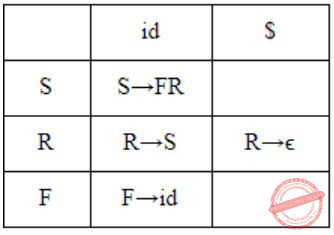The representation M[X,Y] means X represents Variable (rows) and Y represents terminals (columns).
The productions are filled in parsing table by the below mentioned rules:
For every production P → α, we have:
Rule 1: If P → α is a production then add this production for each terminal “t” which is in FIRST of [α] i.e., ADD P → α to M[P, a]
Rule 2: If “ϵ” belongs to FIRST of [P] then add P → α to M[P, b] where “b” represents terminals FOLLOW[P].
By the above rules, we can see that production S → FR will go M[S, a] where “a” is FIRST [FR] which is equal to FIRST[F] = id, So S → FR will go in M[S,id].
Since in the production R→ϵ , FIRST[ϵ] = ϵ, hence the production will go in M[R, b] where “b” represents terminals FOLLOW[R] and FOLLOW[R] = \$, so production R→ϵ will go in M[R,\$]
 Question 59

Consider the following translation scheme.

```   S → ER
R → *E{print("*");}R|ε
E → F + E {print("+");}|F
F → (S)|id {print(id.value);} ```

Here id is a token that represents an integer and id.value represents the corresponding integer value. For an input '2 * 3 + 4', this translation scheme prints

 A 2 * 3 + 4 B 2 * +3 4 C 2 3 * 4 + D 2 3 4+*
Compiler-Design       Syntax-Directed-Translation
Question 59 Explanation: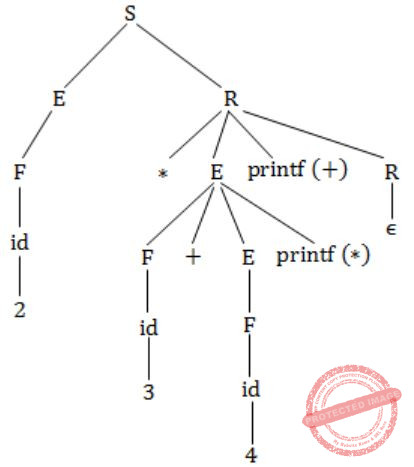Now perform post order evaluation, you will get output as,
2 3 4 + *
 Question 60

Consider the following C code segment.

```for (i = 0, i < n; i++)
{
for (j = 0; j < n; j++)
{
if (i%2)
{
x += (4*j + 5*i);
y += (7 + 4*j);
}
}
}```

Which one of the following is false?

 A The code contains loop invariant computation B There is scope of common sub-expression elimination in this code C There is scope of strength reduction in this code D There is scope of dead code elimination in this code
Compiler-Design       Code-Optimization
Question 60 Explanation:
→ 4*j is a common sub-expression. So there is a scope of elimination. So B is correct.
→ 5*i can be moved out of inner loop. So can be i%2, here loop invariant computation can be done, so option A is correct.
→ 4*i, 5*j can also be replaced so there is a scope of strength reduction. So C is true.
→ But there is no dead code to eliminate, we can replace the variable representation only.
 Question 61

The atomic fetch-and-set x, y instruction unconditionally sets the memory location x to 1 and fetches the old value of x n y without allowing any intervening access to the memory location x. consider the following implementation of P and V functions on a binary semaphore S.

```void P (binary_semaphore *s)
{
unsigned y;
unsigned *x = &(s->value);
do
{
fetch-and-set x, y;
}
while (y);
}
void V (binary_semaphore *s)
{
S->value = 0;
} ```

Which one of the following is true?

 A The implementation may not work if context switching is disabled in P B Instead of using fetch-and-set, a pair of normal load/store can be used C The implementation of V is wrong D The implementation of V is wrong
Operating-Systems       Process-Synchronization
Question 61 Explanation:
A) This is correct because implementation might not work if context switching is disabled in P, then process which is currently blocked may never give control to the process which might eventually execute v. So context switching is must.
B) If we use normal load and store instead of Fetch and Set, then there can be chance that more than one process sees S.value as 0 and then mutual exclusion will not be satisfied. So wrong.
C) Here we are setting S→value to 0, which is correct. This option that's why wrong.
D) Only one process can be in critical section at any time. So this option is wrong.
 Question 62

A CPU generates 32-bit virtual addresses. The page size is 4 KB. The processor has a translation look-aside buffer (TLB) which can hold a total of 128 page table entries and is 4-way set associative. The minimum size of the TLB tag is:

 A 11 bits B 13 bits C 15 bits D 20 bits
Operating-Systems       Virtual Memory
Question 62 Explanation:
Page size = 4 KB = 4 × 210 Bytes = 212 Bytes
Virtual Address = 32 bit
No. of bits needed to address the page frame = 32 - 12 = 20
TLB can hold 128 page table entries with 4-way set associative
⇒ 128/4=32=25
→ 5 bits are needed to address a set.
→ The size of TLB tag = 20 - 5 = 15 bits
 Question 63

A computer system supports 32-bit virtual addresses as well as 32-bit physical addresses. Since the virtual address space is of the same size as the physical address space, the operating system designers decide to get rid of the virtual memory entirely. Which one of the following is true?

 A Efficient implementation of multi-user support is no longer possible B The processor cache organization can be made more efficient now C Hardware support for memory management is no longer needed D CPU scheduling can be made more efficient now
Operating-Systems       Virtual Memory
Question 63 Explanation:
→ When designer decides to get rid of virtual memory entirely then hardware support is no longer needed.
→ Because special hardware support needed only for virtual memory.
 Question 64

Consider three processes (process id 0, 1, 2 respectively) with compute time bursts 2, 4 and 8 time units. All processes arrive at time zero. Consider the longest remaining time first (LRTF) scheduling algorithm. In LRTF ties are broken by giving priority to the process with the lowest process id. The average turnaround time is:

 A 13 units B 14 units C 15 units D 16 units
Operating-Systems       Process-Scheduling
Question 64 Explanation:
Algorithm: LRTF (Longest Remaining Time First)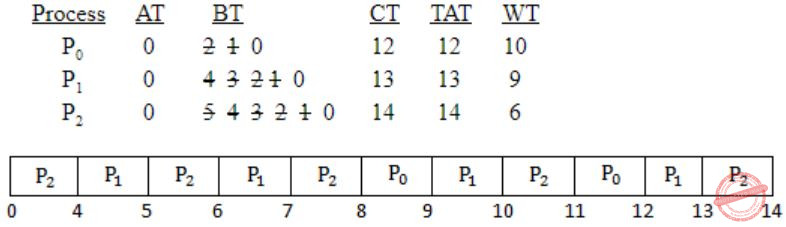Avg TAT = 12+13+14/3 = 39/3 = 13 units
 Question 65

Consider three processes, all arriving at time zero, with total execution time of 10, 20 and 30 units, respectively. Each process spends the first 20% of execution time doing I/O, the next 70% of time doing computation, and the last 10% of time doing I/O again. The operating system uses a shortest remaining compute time first scheduling algorithm and schedules a new process either when the running process gets blocked on I/O or when the running process finishes its compute burst. Assume that all I/O operations can be overlapped as much as possible. For what percentage of time does the CPU remain idle?

 A 0% B 10.6% C 30.0% D 89.4%
Operating-Systems       Process-Scheduling
Question 65 Explanation:Total time needed to complete the execution = 47
Idle time = 2+3 = 5
Percentage of Idle time = 5/47 × 100 =10.6%
 Question 66

Consider the following snapshot of a system running n processes. Process i is holding xi instances of a resource R, 1≤i≤n. Currently, all instances of R are occupied. Further, for all i, process i has placed a request for an additional yi instances while holding the xi instances it already has. There are exactly two processes p and q such that yp = yq = 0. Which one of the following can serve as a necessary condition to guarantee that the system is not approaching a deadlock?

 A min (xp, xq) < maxk≠p,qyk B xp + xq ≥ mink≠p,qyk C max (xp, xq) > 1 D min (xp, xq) > 1
Operating-Systems       Deadlock
Question 66 Explanation:
Deadlock refers stops the execution of process due to non-availability of resources.
→ When two (or) more processes waiting for another process to release the resources.
→ P and Q can execute if they have sufficient resources, they don’t wait for extra resources (i.e., Xp+ Xq) required.
→ Option B can satisfies the corresponding equation i.e., Xp+ Xq >= min(Yk) where k != p and k != q.
Here we have sufficient resources.
 Question 67

Consider the relation account (customer, balance) where customer is a primary key and there are no null values. We would like to rank customers according to decreasing balance. The customer with the largest balance gets rank 1. ties are not broke but ranks are skipped: if exactly two customers have the largest balance they each get rank 1 and rank 2 is not assigned.

```Query1:
select A.customer, count(B.customer)
from account A, account B
where A.balance <=B.balance
group by A.customer

Query2:
select A.customer, 1+count(B.customer)
from account A, account B
where A.balance < B.balance
group by A.customer```

Consider these statements about Query1 and Query2.

```1. Query1 will produce the same row set as Query2 for
some but not all databases.
2. Both Query1 and Query2 are correct implementation
of the specification
3. Query1 is a correct implementation of the specification
but Query2 is not
4. Neither Query1 nor Query2 is a correct implementation
of the specification
5. Assigning rank with a pure relational query takes
less time than scanning in decreasing balance order
assigning ranks using ODBC.```

Which two of the above statements are correct?

 A 2 and 5 B 1 and 3 C 1 and 4 D 3 and 5
Database-Management-System       SQL
Question 67 Explanation:
Query 1 & 2 gives the same output for all not all data based its true because the salaries may be distinct variables. Statement 1 is true.
The customer with largest balance gets rank 1. Ties are broken with ranks are skipped.
So, both queries may doesn’t give same output. Statement 4 is correct.
 Question 68

Consider the relation "enrolled(student, course)" in which (student, course) is the primary key, and the relation "paid(student, amount)" where student is the primary key. Assume no null values and no foreign keys or integrity constraints. Given the following four queries:

```Query1: select student from enrolled where student in (select student from paid)
Query2: select student from paid where student in (select student from enrolled)
Query3: select E.student from enrolled E, paid P where E.student = P.student
Query4: select student from paid where exists
(select * from enrolled where enrolled.student = paid.student)```

Which one of the following statements is correct?

 A All queries return identical row sets for any database. B Query2 and Query4 return identical row sets for all databases but there exist databases for which Query1 and Query2 return different row sets. C There exist databases for which Query3 returns strictly fewer rows than Query2. D There exist databases for which Query4 will encounter an integrity violation at runtime.
Database-Management-System       SQL
Question 68 Explanation:
Consider Table examples as:Query1 : Output
abcd
abcd
PQRS
Query 2 : Output
abcd
PQRS
Query 3 : Output
abcd
PQRS
Query 4 : Output
abcd
PQRS
Query 2 & Query 4 gives same results but Query 1 & Query 3 gives different results.
 Question 69

Consider the relation enrolled(student, course) in which (student, course) is the primary key, and the relation paid(student, amount), where student is the primary key. Assume no null values and no foreign keys or integrity constraints. Assume that amounts 6000, 7000, 8000, 9000 and 10000 were each paid by 20% of the students. Consider these query plans (Plan 1 on left, Plan 2 on right) to "list all courses taken by students who have paid more than x".A disk seek takes 4ms, disk data transfer bandwidth is 300 MB/s and checking a tuple to see if amount is greater than x takes 10 micro-seconds. Which of the following statements is correct?

 A Plan 1 and Plan 2 will not output identical row sets for all databases. B A course may be listed more than once in the output of Plan 1 for some databases. C For x = 5000, Plan 1 executes faster than Plan 2 for all databases. D For x = 9000, Plan I executes slower than Plan 2 for all databases.
Database-Management-System       SQL
Question 69 Explanation:
Both plans are require the tables such as courses and enrolled to access the disks takes same time for both plans.
While analyze the plan1 and plan2 does lesser number of comparisons compared to plan1.
i) The join table consists of two tables will have more rows. So comparisons are needed to find amount greater than x.
ii) Join operation consists of more number of comparisons as the second table will have more rows in plan2 compared to plan1.
 Question 70

The following functional dependencies are given:

`  AB → CD, AF → D, DE → F, C → G, F → E, G → A.  `

Which one of the following options is false?

 A {CF}+ = {ACDEFG} B {BG}+ = {ABCDG} C {AF}+ = {ACDEFG} D {AB}+ = {ABCDFG}
Database-Management-System       Functional-Dependency
Question 70 Explanation:
AB → CD
AF → D
DE → F
C → G
F → E
G → A
CF+ = {G,E,A,D,C,F} = {A,C,D,E,F,G} (✔️)
BG+ = {B,G,A,C,D} = {A,B,C,D,G} (✔️)
AF+ = {D,E,A,F} = {A,D,E,F} (❌)
AB+ = {A,B,C,D,G} (❌)
 Question 71

The 2n vertices of a graph G corresponds to all subsets of a set of size n, for n ≥ 6. Two vertices of G are adjacent if and only if the corresponding sets intersect in exactly two elements.

The number of vertices of degree zero in G is:

 A 1 B n C n+1 D 2n
Engineering-Mathematics       Graph-Theory
Question 71 Explanation:
No. of vertices with degree zero is
= no. of subsets with size less than or equal to 1
= n+1, because in question it is given that the two vertices are connected if and only if the corresponding sets intersect in exactly two elements.
 Question 72

The 2n vertices of a graph G corresponds to all subsets of a set of size n, for n ≥ 6. Two vertices of G are adjacent if and only if the corresponding sets intersect in exactly two elements.

The maximum degree of a vertex in G is:

 AB 2n-2 C 2n-3 × 3 D 2n-1
Engineering-Mathematics       Graph-Theory
Question 72 Explanation:
The degree of each subset with k elements will be
(k(c))2 2n-k
∴ We need to find 'k' value such that, the value will be maximum.[k should be an integer].
If you differentiate (k(c))2 2n-k w.r.t. k and equal to 0.
You will get k = 2/(loge)2 which is not an integer.
So you can see it like∴ The maximum degree 3⋅2n-3 at k=3 or k=4.
 Question 73

The 2n vertices of a graph G corresponds to all subsets of a set of size n, for n ≥ 6. Two vertices of G are adjacent if and only if the corresponding sets intersect in exactly two elements.

The number of connected components in G is:

 A n B n+2 C 2n/2 D 2n / n
Engineering-Mathematics       Graph-Theory
Question 73 Explanation:
Not connected nodes is n+1.
While other nodes are connected so that total number of connected components is (n+1)+1
(here we are adding 1 because it is connected corresponding remaining vertices)
= n+2
 Question 74

Consider two cache organizations: The first one is 32 KB 2-way set associative with 32-byte block size. The second one is of the same size but direct mapped. The size of an address is 32 bits in both cases. A 2-to-1 multiplexer has a latency of 0.6 ns while a kbit comparator has a latency of k/10 ns. The hit latency of the set associative organization is h1 while that of the direct mapped one is h2.

The value of h1 is:

 A 2.4 ns B 2.3 ns C 1.8 ns D 1.7 ns
Computer-Organization       Cache
Question 74 Explanation:
Cache size = 32 KB = 32 × 210 B
Block size = 32 bytes
No. of blocks = 2 (2-way set associative)
No. of combinations = Cache size / (Block size×No. of blocks) = (32×210B) / (32×2) = 29
Index bits = 9
No. of set bits = 5 (∵ cache block size is 32 bytes = 25 bytes)
No. of Tag bits = 32 - 9 - 5 = 18
Hit latency = Multiplexer latency + latency
= 0.6 + (18/10)
= 0.6 + 1.8
= 2.4 ns
 Question 75

Consider two cache organizations: The first one is 32 KB 2-way set associative with 32-byte block size. The second one is of the same size but direct mapped. The size of an address is 32 bits in both cases. A 2-to-1 multiplexer has a latency of 0.6 ns while a kbit comparator has a latency of k/10 ns. The hit latency of the set associative organization is h1 while that of the direct mapped one is h2.

The value of h2 is:

 A 2.4 ns B 2.3 ns C 1.8 ns D 1.7 ns
Computer-Organization       Cache
Question 75 Explanation:
Hit latency = k/10 ns
For k,∴ Hit latency = 17/1 = 1.7 ns
 Question 76

A 3-ary max heap is like a binary max heap, but instead of 2 children, nodes have 3 children. A 3-ary heap can be represented by an array as follows: The root is stored in the first location, a, nodes in the next level, from left to right, is stored from a to a. The nodes from the second level of the tree from left to right are stored from a location onward. An item x can be inserted into a 3-ary heap containing n items by placing x in the location a[n] and pushing it up the tree to satisfy the heap property.

Which one of the following is a valid sequence of elements in an array representing 3-ary max heap?

 A 1, 3, 5, 6, 8, 9 B 9, 6, 3, 1, 8, 5 C 9, 3, 6, 8, 5, 1 D 9, 5, 6, 8, 3, 1
Data-Structures       Heap-Tree
Question 76 Explanation:Question 77

A 3-ary max heap is like a binary max heap, but instead of 2 children, nodes have 3 children. A 3-ary heap can be represented by an array as follows: The root is stored in the first location, a, nodes in the next level, from left to right, is stored from a to a. The nodes from the second level of the tree from left to right are stored from a location onward. An item x can be inserted into a 3-ary heap containing n items by placing x in the location a[n] and pushing it up the tree to satisfy the heap property.

Suppose the elements 7, 2, 10 and 4 are inserted, in that order, into the valid 3-ary max heap found in the above question, Q.76. Which one of the following is the sequence of items in the array representing the resultant heap?

 A 10, 7, 9, 8, 3, 1, 5, 2, 6, 4 B 10, 9, 8, 7, 6, 5, 4, 3, 2, 1 C 10, 9, 4, 5, 7, 6, 8, 2, 1, 3 D 10, 8, 6, 9, 7, 2, 3, 4, 1, 5
Data-Structures       Heap-Tree
Question 77 Explanation: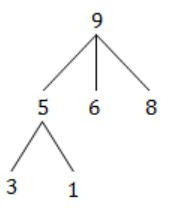Question 78

Barrier is a synchronization construct where a set of processes synchronizes globally i.e. each process in the set arrives at the barrier and waits for all others to arrive and then all processes leave the barrier. Let the number of processes in the set be three and S be a binary semaphore with the usual P and V functions. Consider the following C implementation of a barrier with line numbers shown on left.

```void barrier (void) {
1:   P(S);
2:   process_arrived++;
3.   V(S);
4:   while (process_arrived !=3);
5:   P(S);
6:   process_left++;
7:   if (process_left==3) {
8:      process_arrived = 0;
9:      process_left = 0;
10:  }
11:  V(S);
} ```

The variables process_arrived and process_left are shared among all processes and are initialized to zero. In a concurrent program all the three processes call the barrier function when they need to synchronize globally.

The above implementation of barrier is incorrect. Which one of the following is true?

 A The barrier implementation is wrong due to the use of binary semaphore S B The barrier implementation may lead to a deadlock if two barriers in invocations are used in immediate succession C Lines 6 to 10 need not be inside a critical section D The barrier implementation is correct if there are only two processes instead of three
Operating-Systems       Process-Synchronization
Question 78 Explanation:
If process-arrived is because greater than 3. Then there is no possibility to be 3.
Hence, it is leads to deadlock.
 Question 79

Barrier is a synchronization construct where a set of processes synchronizes globally i.e. each process in the set arrives at the barrier and waits for all others to arrive and then all processes leave the barrier. Let the number of processes in the set be three and S be a binary semaphore with the usual P and V functions. Consider the following C implementation of a barrier with line numbers shown on left.

```void barrier (void) {
1:   P(S);
2:   process_arrived++;
3.   V(S);
4:   while (process_arrived !=3);
5:   P(S);
6:   process_left++;
7:   if (process_left==3) {
8:      process_arrived = 0;
9:      process_left = 0;
10:  }
11:  V(S);
} ```

The variables process_arrived and process_left are shared among all processes and are initialized to zero. In a concurrent program all the three processes call the barrier function when they need to synchronize globally.

Which one of the following rectifies the problem in the implementation?

 A Lines 6 to 10 are simply replaced by process_arrived-- B At the beginning of the barrier the first process to enter the barrier waits until process_arrived becomes zero before proceeding to execute P(S). C Context switch is disabled at the beginning of the barrier and re-enabled at the end. D The variable process_left is made private instead of shared.
Operating-Systems       Process-Synchronization
Question 79 Explanation:
If process-arrived is becomes zero then process_left becomes zero. Then deadlock may resolves.
 Question 80

A CPU has a 32 KB direct mapped cache with 128-byte block size. Suppose A is a two-dimensional array of size 512×512 with elements that occupy 8-bytes each. Consider the following two C code segments, P1 and P2.

```P1: for (i=0; i<512; i++) {
for (j=0; j<512; j++) {
x += A[i][j];
}
}

P2: for (i=0; i<512; i++) {
for (j=0; j<512; j++) {
x += A[j][i];
}
} ```

P1 and P2 are executed independently with the same initial state, namely, the array A is not in the cache and i, j, x are in registers. Let the number of cache misses experienced by P1 be M1 and that for P2 be M2.

The value of M1 is:

 A 0 B 2048 C 16384 D 262144
Computer-Organization       Cache
Question 80 Explanation:
[P1] Access A in row major order.
[P2] Access A in column major order.
No. of cache blocks=Cache size/Block size = 32KB / 128B = 32×210B / 128B = 215 / 27 = 256
No. of array elements in each block = Block size / Element size = 128B / 8B = 16
Total misses for (P1) = Array size * No. of array elements / No. of cache blocks = (512×512) * 16 / 256 = 16384
 Question 81

A CPU has a 32 KB direct mapped cache with 128-byte block size. Suppose A is a two-dimensional array of size 512×512 with elements that occupy 8-bytes each. Consider the following two C code segments, P1 and P2.

```P1: for (i=0; i<512; i++) {
for (j=0; j<512; j++) {
x += A[i][j];
}
}

P2: for (i=0; i<512; i++) {
for (j=0; j<512; j++) {
x += A[j][i];
}
} ```

P1 and P2 are executed independently with the same initial state, namely, the array A is not in the cache and i, j, x are in registers. Let the number of cache misses experienced by P1 be M1 and that for P2 be M2.

The value of the ratio M1/M2 is:

 A 0 B 1/16 C 1/8 D 16
Computer-Organization       Cache
Question 81 Explanation:
Total misses for [P2] = 512 * 512 = 262144
(for every element there would be a miss)
M1/M2 = 16384/262144 = 1/16
 Question 82

Consider the diagram shown below where a number of LANs are connected by (transparent) bridges. In order to avoid packets looping through circuits in the graph, the bridges organize themselves in a spanning tree. First, the root bridge is identified as the bridge with the least serial number. Next, the root sends out (one or more) data units to enable the setting up of the spanning tree of shortest paths from the root bridge to each bridge.

Each bridge identifies a port (the root port) through which it will forward frames to the root bridge. Port conflicts are always resolved in favour of the port with the lower index value. When there is a possibility of multiple bridges forwarding to the same LAN (but not through the root port), ties are broken as follows: bridges closest to the root get preference and between such bridges, the one with the lowest serial number is preferred.For the given connection of LANs by bridges, which one of the following choices represents the depth first traversal of the spanning tree of bridges?

 A B1, B5, B3, B4, B2 B B1, B3, B5, B2, B4 C B1, B5, B2, B3, B4 D B1, B3, B4, B5, B2
Computer-Networks       Bridges
Question 82 Explanation:
Start Depth First traversal from B1.
B5 is connected to port 1 of B1 so B5 will be traversed after B1.
Port1 of B5 is connected to port1 of B2 and port2 of B3.
B2 is connected with lower index port so B2 is traversed next.
Port 2 Both B3 and B4 is connected with port1 of B2, but B3 is Closer to root so B3 will be traversed next.
Depth First traversal is B1, B5, B2, B3, B4.
 Question 83

Consider the diagram shown below where a number of LANs are connected by (transparent) bridges. In order to avoid packets looping through circuits in the graph, the bridges organize themselves in a spanning tree. First, the root bridge is identified as the bridge with the least serial number. Next, the root sends out (one or more) data units to enable the setting up of the spanning tree of shortest paths from the root bridge to each bridge.

Each bridge identifies a port (the root port) through which it will forward frames to the root bridge. Port conflicts are always resolved in favour of the port with the lower index value. When there is a possibility of multiple bridges forwarding to the same LAN (but not through the root port), ties are broken as follows: bridges closest to the root get preference and between such bridges, the one with the lowest serial number is preferred.Consider the correct spanning tree for the previous question. Let host H1 send out a broadcast ping packet. Which of the following options represents the correct forwarding table on B3?

 A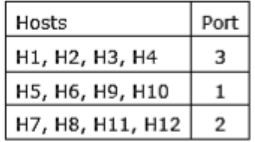BC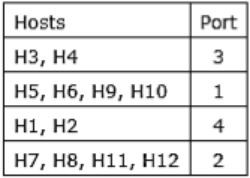D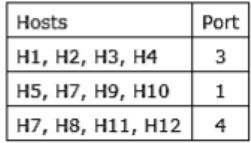Computer-Networks       Bridges
Question 83 Explanation:
Use Spanning tree generated in previous question.
 Question 84

Which one of the following grammars generates the language L = {aibj|i≠j}?

 A S→AC|CB C→aCb|a|b A→aA|ϵ B→Bb|ϵ B S→aS|Sb|a|b C S→AC|CB C→aCb|ϵ A→aA|ϵ B→Bb|ϵ D S→AC|CB C→aCb|ϵ A→aA|a B→Bb|b
Theory-of-Computation       Grammar
Question 84 Explanation:
The language have all the strings in which a’s comes before b’s and number of a’s never equal to b’s. The grammars in Option A, B and C generates string “ab” in which number of a’s are equal to b’s, hence they are wrong. But option D generates all the string which is in L.
 Question 85

In the correct grammar of above question, what is the length of the derivation (number of steps starting from S) to generate the string albm with l≠m?

 A max(l,m)+2 B l+m+2 C l+m+3 D max(l, m)+3
Theory-of-Computation       Grammar
Question 85 Explanation:
The correct grammar for L = {aibj | i≠j} is
S → AC|CB C → aCb|ϵ A → aA|a B → Bb|b
Assume a string: “aaabb” in this l=3 and m=2
The steps are:
Step1: S-> AC
Step 2: S-> aC By production: A-> a
Step 3: S-> aaCb By production: C-> aCb
Step 4: S-> aaaCbb By production: C-> aCb
Step 5: S-> aaabb By production: C-> ϵ
Hence, it is clear that the correct option is A, i.e. max(l,m)+2
There are 85 questions to complete.
PHP Code Snippets Powered By : XYZScripts.com
error: Content is protected !!